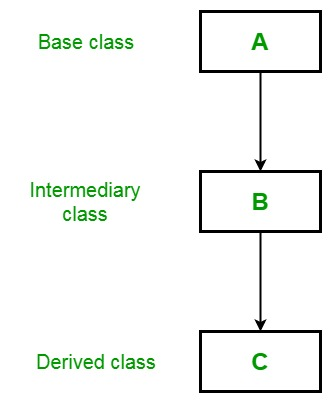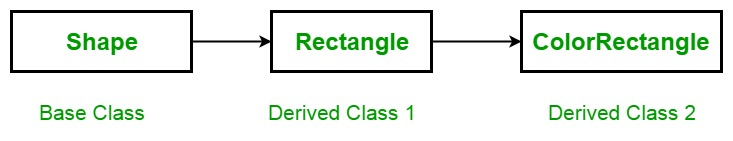Open in App
Not now

# C# | Multilevel Inheritance

• Difficulty Level : Medium
• Last Updated : 23 Jan, 2019

In the Multilevel inheritance, a derived class will inherit a base class and as well as the derived class also act as the base class to other class. For example, three classes called A, B, and C, as shown in the below image, where class C is derived from class B and class B, is derived from class A. In this situation, each derived class inherit all the characteristics of its base classes. So class C inherits all the features of class A and B.Example: Here, the derived class Rectangle is used as a base class to create the derived class called ColorRectangle. Due to inheritance the ColorRectangle inherit all the characteristics of Rectangle and Shape and add an extra field called rcolor, which contains the color of the rectangle.This example also covers the concept of constructors in a derived class. As we know that a subclass inherits all the members (fields, methods) from its superclass but constructors are not members, so they are not inherited by subclasses, but the constructor of the superclass can be invoked from the subclass. As shown in the below example, base refers to a constructor in the closest base class. The base in ColorRectangle calls the constructor in Rectangle and the base in Rectangle class the constructor in Shape.

 `// C# program to illustrate the ` `// concept of multilevel inheritance ` `using` `System; ` ` `  `class` `Shape { ` ` `  `    ``double` `a_width; ` `    ``double` `a_length; ` ` `  `    ``// Default constructor ` `    ``public` `Shape() ` `    ``{ ` `        ``Width = Length = 0.0; ` `    ``} ` ` `  `    ``// Constructor for Shape ` `    ``public` `Shape(``double` `w, ``double` `l) ` `    ``{ ` `        ``Width = w; ` `        ``Length = l; ` `    ``} ` ` `  `    ``// Construct an object with  ` `    ``// equal length and width ` `    ``public` `Shape(``double` `y) ` `    ``{ ` `        ``Width = Length = y; ` `    ``} ` ` `  `    ``// Properties for Length and Width ` `    ``public` `double` `Width ` `    ``{ ` `        ``get` `{ ` `               ``return` `a_width;  ` `            ``} ` ` `  `        ``set` `{  ` `              ``a_width = value < 0 ? -value : value;  ` `            ``} ` `    ``} ` ` `  `    ``public` `double` `Length ` `    ``{ ` `        ``get` `{  ` `               ``return` `a_length;  ` `            ``} ` ` `  `        ``set` `{  ` `              ``a_length = value < 0 ? -value : value; ` `            ``} ` `    ``} ` `    ``public` `void` `DisplayDim() ` `    ``{ ` `        ``Console.WriteLine(``"Width and Length are "`  `                     ``+ Width + ``" and "` `+ Length); ` `    ``} ` `} ` ` `  `// A derived class of Shape  ` `// for the rectangle. ` `class` `Rectangle : Shape { ` ` `  `    ``string` `Style; ` ` `  `    ``// A default constructor.  ` `    ``// This invokes the default ` `    ``// constructor of Shape. ` `    ``public` `Rectangle() ` `    ``{ ` `        ``Style = ``"null"``; ` `    ``} ` ` `  `    ``// Constructor ` `    ``public` `Rectangle(``string` `s, ``double` `w, ``double` `l) ` `        ``: ``base``(w, l) ` `    ``{ ` `        ``Style = s; ` `    ``} ` ` `  `    ``// Construct an square. ` `    ``public` `Rectangle(``double` `y) ` `        ``: ``base``(y) ` `    ``{ ` `        ``Style = ``"square"``; ` `    ``} ` ` `  `    ``// Return area of rectangle. ` `    ``public` `double` `Area() ` `    ``{ ` `        ``return` `Width * Length; ` `    ``} ` ` `  `    ``// Display a rectangle's style. ` `    ``public` `void` `DisplayStyle() ` `    ``{ ` `        ``Console.WriteLine(``"Rectangle is  "` `+ Style); ` `    ``} ` `} ` ` `  `// Inheriting Rectangle class ` `class` `ColorRectangle : Rectangle { ` ` `  `    ``string` `rcolor; ` ` `  `    ``// Constructor ` `    ``public` `ColorRectangle(``string` `c, ``string` `s, ` `                          ``double` `w, ``double` `l) ` `        ``: ``base``(s, w, l) ` `    ``{ ` `        ``rcolor = c; ` `    ``} ` ` `  `    ``// Display the color. ` `    ``public` `void` `DisplayColor() ` `    ``{ ` `        ``Console.WriteLine(``"Color is "` `+ rcolor); ` `    ``} ` `} ` ` `  `// Driver Class ` `class` `GFG { ` ` `  `    ``// Main Method ` `    ``static` `void` `Main() ` `    ``{ ` `        ``ColorRectangle r1 = ``new` `ColorRectangle(``"pink"``,  ` `                   ``"Fibonacci rectangle"``, 2.0, 3.236); ` ` `  `        ``ColorRectangle r2 = ``new` `ColorRectangle(``"black"``, ` `                                   ``"Square"``, 4.0, 4.0); ` ` `  `        ``Console.WriteLine(``"Details of r1: "``); ` `        ``r1.DisplayStyle(); ` `        ``r1.DisplayDim(); ` `        ``r1.DisplayColor(); ` ` `  `        ``Console.WriteLine(``"Area is "` `+ r1.Area()); ` `        ``Console.WriteLine(); ` ` `  `        ``Console.WriteLine(``"Details of r2: "``); ` `        ``r2.DisplayStyle(); ` `        ``r2.DisplayDim(); ` `        ``r2.DisplayColor(); ` ` `  `        ``Console.WriteLine(``"Area is "` `+ r2.Area()); ` `    ``} ` `} `

Output:

```Details of r1:
Rectangle is  Fibonacci rectangle
Width and Length are 2 and 3.236
Color is pink
Area is 6.472

Details of r2:
Rectangle is  Square
Width and Length are 4 and 4
Color is black
Area is 16
```

My Personal Notes arrow_drop_up
Related Articles